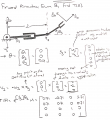# Forward Kinematics on MATLAB

#### kucar17

Joined Apr 26, 2020
7
Hello there, hope you are all well.

I am taking a Robotics Course on Coursera and studying Forward Kinematics right now. I wanted to try to create a Transformation Matrix on MATLAB for a end-effector frame of a robotic arm.This is the Robotic Arm, and at the below you can see the transformation Matrix, if q3 is rotated 45 degrees about the z axis.

Here is my code and its output:

Code:
clc
clear
omega3 = [0 0 1]';
v3 = [0 -2 0]';
omega3Bracket = [0, -omega3(3), omega3(2); omega3(3), 0, -omega3(1); -omega3(2), omega3(1), 0];
S3Bracket = [omega3Bracket, v3; 0 0 0 0];
expm(S3Bracket.*pi/4)
On the third and fourth lines, I created the angular and linear velocity vectors.

On the fifth line, I converted the angular velocity vector to a 3x3 skew-symmetric matrix.

On the sixth line, I created the skew-symmetric Screw matrix.

On the seventh line, I used matrix exponentilal to calculate the Transformation Matrix. However, the last column of the matrix I got is different than the one I should be able to get. Dou you know the reason foe that? What am I doing wrong?

Output:

Code:
    0.7071   -0.7071         0    0.5858
0.7071    0.7071         0   -1.4142
0         0    1.0000         0
0         0         0    1.0000